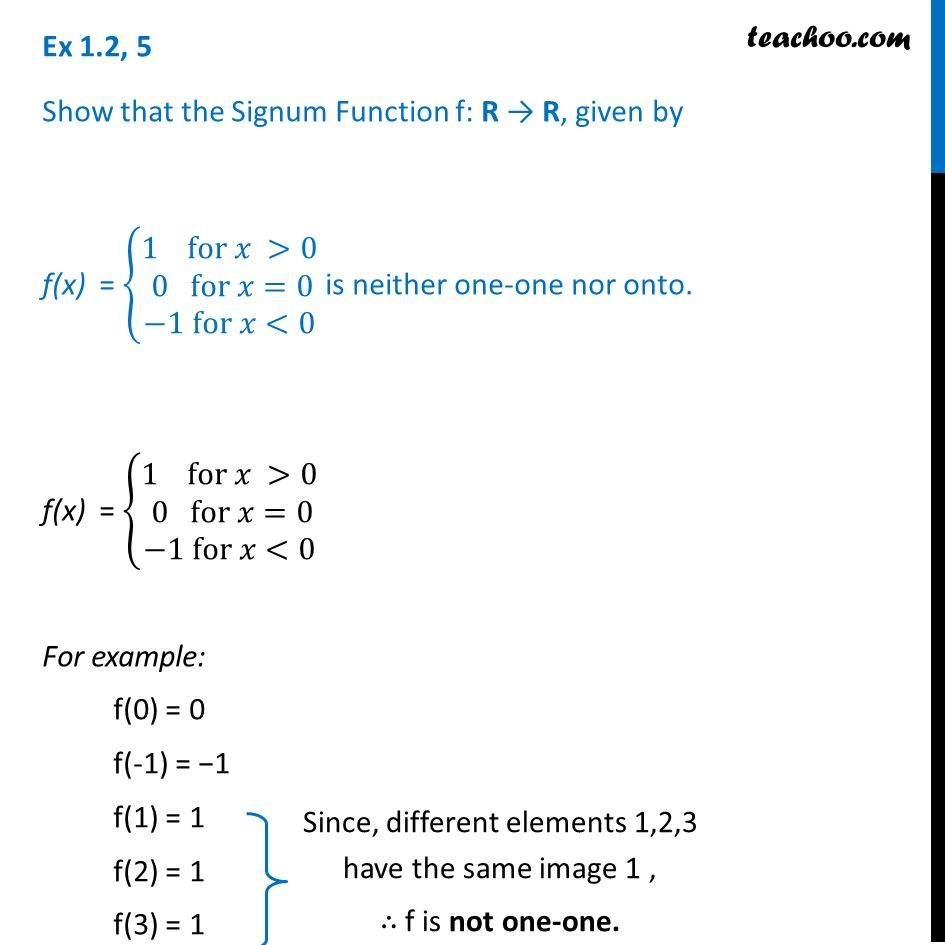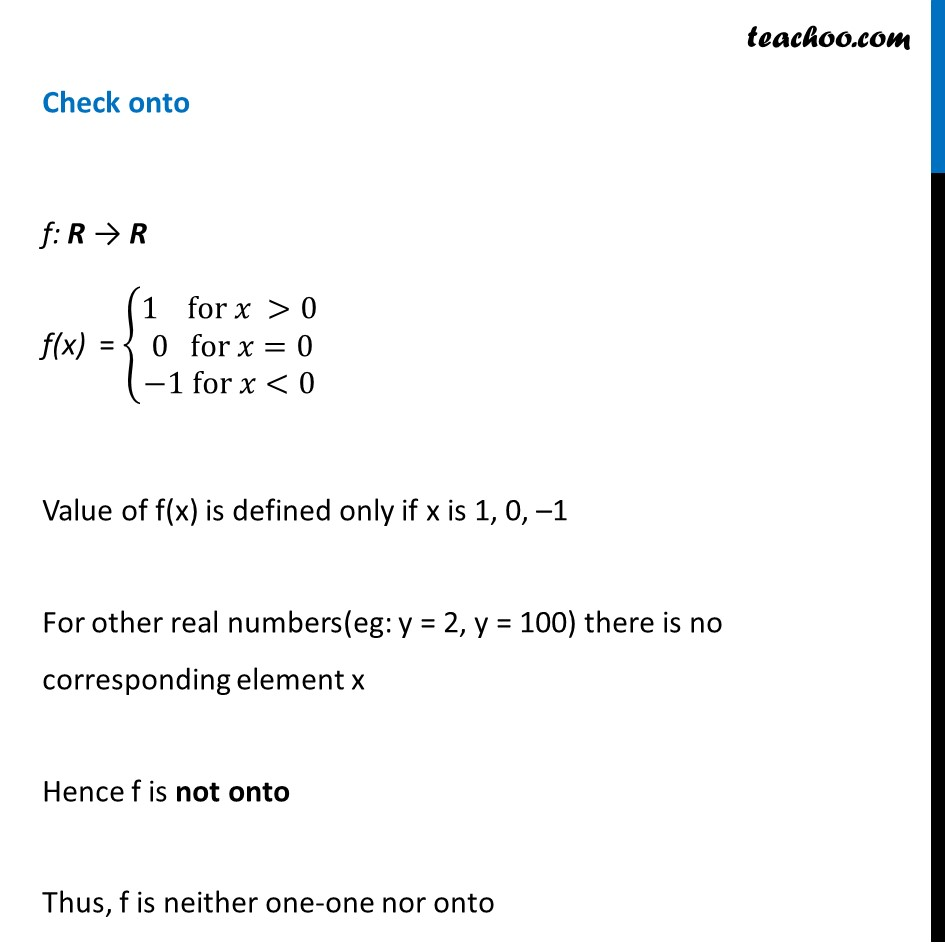Chapter 1 Class 12 Relation and Functions

Class 12
Important Questions for exams Class 12Learn in your speed, with individual attention - Teachoo Maths 1-on-1 Class

### Transcript

Ex 1.2, 5 Show that the Signum Function f: R → R, given by f(x) = {█(1 for 𝑥 >0@ 0 for 𝑥=0@−1 for 𝑥<0)┤ is neither one-one nor onto. f(x) = {█(1 for 𝑥 >0@ 0 for 𝑥=0@−1 for 𝑥<0)┤ For example: f(0) = 0 f(-1) = −1 f(1) = 1 f(2) = 1 f(3) = 1 Since, different elements 1,2,3 have the same image 1 , ∴ f is not one-one. Check onto f: R → R f(x) = {█(1 for 𝑥 >0@ 0 for 𝑥=0@−1 for 𝑥<0)┤ Value of f(x) is defined only if x is 1, 0, –1 For other real numbers(eg: y = 2, y = 100) there is no corresponding element x Hence f is not onto Thus, f is neither one-one nor onto